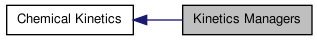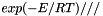# Kinetics Managers [Chemical Kinetics]

Collaboration diagram for Kinetics Managers:## Classes

class  Kinetics
Public interface for kinetics managers. More...

## Models and Managers

A kinetics manager is a C++ class that implements a kinetics model; a kinetics model is a set of mathematical equation describing how various kinetic quanities are to be computed -- reaction rates, species production rates, etc. Many different kinetics models might be defined to handle different types of kinetic processes. For example, one kinetics model might use expressions valid for elementary reactions in ideal gas mixtures. It might, for example, require the reaction orders to be integral and equal to the forward stoichiometric coefficients, require that each reaction be reversible with a reverse rate satisfying detailed balance, include pressure-dependent unimolecular reactions, etc. Another kinetics model might be designed for heterogeneous chemistry at interfaces, and might allow empirical reaction orders, coverage-dependent activation energies, irreversible reactions, and include effects of potential differences across the interface on reaction rates.

A kinetics manager implements a kinetics model. Since the model equations may be complex and expensive to evaluate, a kinetics manager may adopt various strategies to 'manage' the computation and evaluate the expressions efficiently. For example, if there are rate coefficients or other quantities that depend only on temperature, a manager class may choose to store these quantities internally, and re-evaluate them only when the temperature has actually changed. Or a manager designed for use with reaction mechanisms with a few repeated activation energies might precompute the terms, instead of evaluating the exponential repeatedly for each reaction. There are many other possible 'management styles', each of which might be better suited to some reaction mechanisms than others.

But however a manager structures the internal computation, the tasks the manager class must perform are, for the most part, the same. It must be able to compute reaction rates, species production rates, equilibrium constants, etc. Therefore, all kinetics manager classes should have a common set of public methods, but differ in how they implement these methods.

A kinetics manager computes reaction rates of progress, species production rates, equilibrium constants, and similar quantities for a reaction mechanism. All kinetics manager classes derive from class Kinetics, which defines a common public interface for all kinetics managers. Each derived class overloads the virtual methods of Kinetics to implement a particular kinetics model.

For example, class GasKinetics implements reaction rate expressions appropriate for homogeneous reactions in ideal gas mixtures, and class InterfaceKinetics implements expressions appropriate for heterogeneous mechanisms at interfaces, including how to handle reactions involving charged species of phases with different electric potentials --- something that class GasKinetics doesn't deal with at all.

Kinetics managers may be also created that hard-wire a particular reaction mechanism in C++ code. This can often result in faster performance. An example of this is the kinetics manager GRI30_Kinetics that hard-wires the rate expressions for the natural gas combustion mechanism GRI-3.0.

Many of the methods of class Kinetics write into arrays the values of some quantity for each species, for example the net production rate. These methods always write the results into flat arrays, ordered by phase in the order the phase was added, and within a phase in the order the species were added to the phase (which is the same ordering as in the input file). Example: suppose a heterogeneous mechanism involves three phases -- a bulk phase 'a', another bulk phase 'b', and the surface phase 'a:b' at the a/b interface. Phase 'a' contains 12 species, phase 'b' contains 3, and at the interface there are 5 adsorbed species defined in phase 'a:b'. Then methods like getNetProductionRates(doublereal* net) will write and output array of length 20, beginning at the location pointed to by 'net'. The first 12 values will be the net production rates for all 12 species of phase 'a' (even if some do not participate in the reactions), the next 3 will be for phase 'b', and finally the net production rates for the surface species will occupy the last 5 locations.

Generated by1.6.3